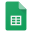# Linear Algebra 1Thailand

## Lectures

Chapter 1Algebraic Prerequisites - PDF

Chapter 2Linear Maps and Endomorphisms - PDF

Chapter 3Polynomials of Matrices - PDF

Chapter 4Diagonalization - PDF

Chapter 5Jordan Normal Form - PDF

Chapter 6Special Matrices - PDF

## Homework

Homework 1 • 2, 4, 6, 8, 10 - Groups (Chapter 1)

Homework 2 • 12, 14, 16, 18, 20 - Groups (Chapter 1)

Homework 3 • 22, 24, 28, 30, 32 - Rings and Fields (Chapter 1)

Homework 4 • 34, 36, 40, 42, 44 - Fields and Polynomials (Chapter 1)

Homework 5 • 1, 2, 4, 6, 8 - Vector Spaces and Subspaces - PDF

Homework 6 • 2, 4, 6, 8, 10 - Linear Maps and Endomorphismes (Chapter 2)

Homework 7 • 22, 24, 26, 30, 32 - Eigenvalues and Eigenspaces (Chapter 2)

Homework 8 • 2, 11, 18, 20, 28 - Polynomials of Matrices (Chapter 3)

Homework 9 • 4, 6, 10, 12, 14 - Diagonalization (Chapter 4)

Homework 10 • 2, 4, 6, 8, 10 - Jordan Normal Form (Chapter 5)

## ResultsResults / Linear Algebra 1 / Thailand

* The percentage of your homework is calculated as the maximum percentage times the ratio of your score to the maximum score

** In-class participation takes into account your class attendance and involvement in the process

## Literature

1. Essential Linear Algebra with Applications: A Problem-Solving Approach, Titu Andreescu, Birkhäuser 2014

2. Schaum's Outline of Linear Algebra, Seymour Lipschutz and Marc Lars Lipson, McGraw-Hill 2009

3. Linear Algebra: A Modern Introduction, David Poole, Cengage Learning 2015